# Writing a simple Compiler on my own - Action Rules for Function Calls [C][Flex][Bison]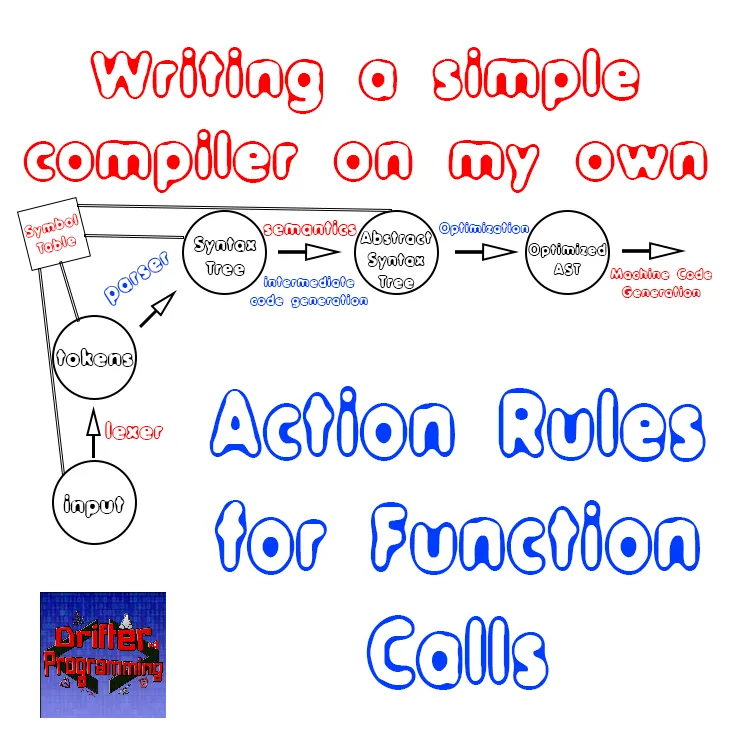[Custom Thumbnail]

All the Code of the series can be found at the Github repository:
https://github.com/drifter1/compiler

# Introduction

Hello it's a me again @drifter1! Today we continue with my Compiler Series, a series where we implement a complete compiler for a simple C-like language by using the C-tools Flex and Bison. In this article we will start writing the needed Action Rules for Function Calls. This is the last article were we will be doing stuff around the AST structure! From next time on we will be extending this structure and the action rules of the Parser to check different kinds of semantics :)

## More specifically, the topics that we will cover today are:

1. Visualizing the Problem
2. AST nodes and creation functions
3. Action Rules for Function Calls
4. Running the compiler

## Requirements:

Actually you need to read and understand all the topics that I covered in the series as a whole, as these articles will give you access to knowledge about:
• What Compiler Design is (mainly the steps)
• For which exact Language the Compiler is build for (Tokens and Grammar)
• How to use Flex and Bison
• How to implement a lexer and parser for the language using those tools
• What the Symbol Table is and how we implement it
• How we combine Flex and Bison together
• How we can pass information from the Lexer to the Parser
• How we define operator priorities, precedencies and associativity
• What Semantic Analysis is (Attributes, SDT etc.)
• How we do the so called "Scope Resolution"
• How we declare types and check the type inter-compatibility for different cases, including function parameters
• How we check function calls later on using a "Revisit queue", as function declarations mostly happen after functions get used (in our Language)
• Intermediate Code Representations, including AST's
• How we implement an AST (structure and management)
• Action Rules for other cases

## Difficulty:

Talking about the series in general this series can be rated:
Today's topic(s) can be rated:
• Medium

# Actual Tutorial Content

## Visualizing the Problem

Let's start out with a visualization of the problem. So, how does a function call look like? Well, we use the name of the function followed by a parenthesis with (calling) parameters separated with commas. The parameters of a function can also be empty, and to allow printing using the function "print" also equal to a constant STRING. When not having a single STRING parameter, the parameters are given in form of any expression!

A function call can be visualized as following: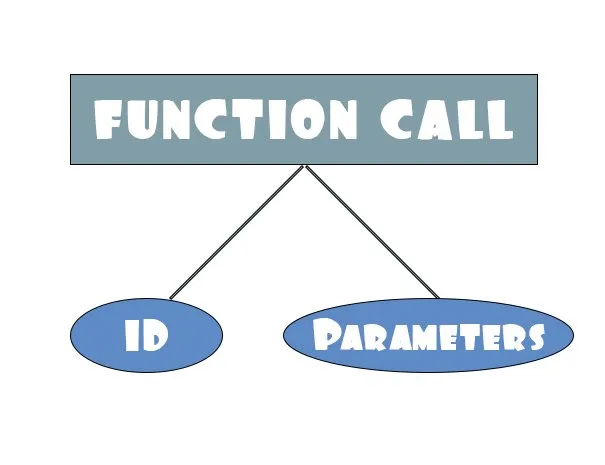Let's separate the parameters into different cases:
• No parameters and so simply func_name()
• STRING parameter and so func_name("example string")
• One parameter in form of an expression func_name(expr)
• Many parameters separated with commas and so func_name(expr, ... , expr)
From these cases the last two can be grouped together to form something similar to the function declaration parameters structure. This structure will contain all the AST Nodes that were used as parameters and their count. So, in the end the different cases of calling parameters look as following: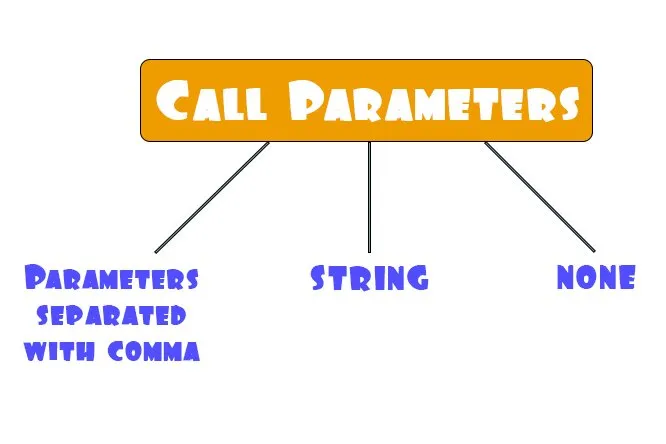The last two are pretty straight forward, even if we will use the same structure as the first one in all of them, to make it easier for us to pass the information over to the function call node! The tree that is formed by function call parameters is of the form: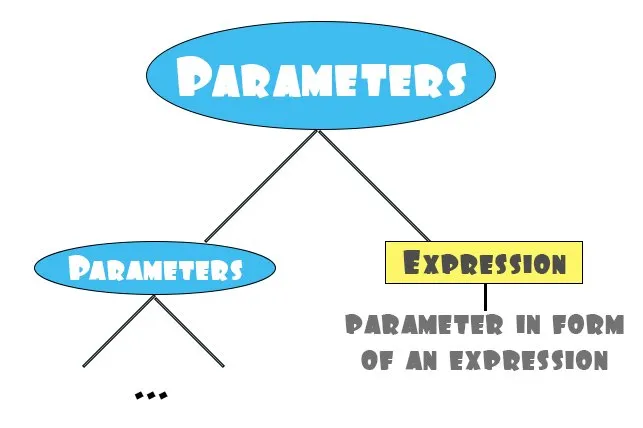So, any call parameters node will contain all the parameters up to this point. We will just add the new parameter to the previous node's array, by also increasing the count of parameter. This "extended" node will then be passed over to the parents node. In the end the last of the parameters nodes will contain all the parameters that occur in the function call! There is no single node type that can be called an "Expression", but there are many different types of them! So, the final array will contain different kinds of expressions. I guess that you can see that all the expressions will have to store their data type, so that we can know if the data type is compatible with the corresponding function declaration parameter. This is what we will be doing next time :)

## AST nodes and creation functions

The whole problem of function calls can therefore be represented by using only two AST Nodes: one for the function call and one for the function call parameters. The second one will only be "temporary". The information of the parameters node will be passed over to the function call node. We already have a function call node, so let's just add a new node type, node and creation function for function call parameters...
The Node_Type enum will have to be extended:
``````typedef enum Node_Type {
...
FUNC_CALL,    // function call
CALL_PARAMS,  // function call parameters
...
}``````

The AST Node structure of function call parameters has to contain an array and a counter. In the end it's just the structure of a function call if we remove the ID entry! To understand it easier it, let's just snip-out both of them:
``````...
typedef struct AST_Node_Func_Call{
enum Node_Type type; // node type
// function identifier
list_t *entry;
// call parameters
AST_Node **params;
int num_of_pars;
}AST_Node_Func_Call;
typedef struct AST_Node_Call_Params{
enum Node_Type type; // node type
// call parameters
AST_Node **params;
int num_of_pars;
}AST_Node_Call_Params;
...``````

As I already mentioned previously a call parameters node will be created using a previously node. So, the creation function of such a node will be similar to the declarations, statements, function declarations and function declaration parameters node! More specifically, we will pass the array and counter of a previous node (or NULL and '0' when there's no previous parameter) and the newly found parameter. Th new parameter will be added to the array and the counter will be incremented. In the end the code looks as following:
``````ast.h:
...
AST_Node *new_ast_call_params_node(AST_Node **params, int num_of_pars, AST_Node *param);
...
---------------------------------------------------------------------------------------
ast.c:
...
AST_Node *new_ast_call_params_node(AST_Node **params, int num_of_pars, AST_Node *param){
// allocate memory
AST_Node_Call_Params *v = malloc (sizeof (AST_Node_Call_Params));
// set type
v->type = CALL_PARAMS;
// first parameter
if(params == NULL){
params = (AST_Node**) malloc (sizeof (AST_Node*));
params = param;
num_of_pars = 1;
}
else{
params = (AST_Node**) realloc (params, (num_of_pars + 1) * sizeof (AST_Node*));
params[num_of_pars] = param;
num_of_pars++;
}
// set entries
v->params = params;
v->num_of_pars = num_of_pars;
// return type-casted result
return (struct AST_Node *) v;
}
...``````

Of course I also made changes in the "ast_print_node" and "ast_traversal" functions, but these are pretty simple changes for the console printing purposes by the end of the article. You can check out the new code for that on GitHub :)

## Action Rules for Function Calls

So, let's now finally use the nodes in the parser's action rules. Function calls occur both as statements and expressions and so the first thing that we have to do is to "push" the information over to "statement" and "expression":
``````statement:
... other-cases ...
| function_call SEMI
{
\$\$ = \$1; /* just pass information */
}
... other-cases ...
;
...
expression:
... other-cases ...
| function_call
{
\$\$ = \$1; /* just pass information */
}
;``````

There are three rules (or non-terminals) that have to do with function calls. All of them store the information in form of an AST Node, which means that we have to include the following non-terminal type definition:
`%type <node> function_call call_params call_param`

Let's get into each of them!

### call_param rule

A call parameters node adds the newly found parameter (in form of an expression) to the previously existing ones of the "child" parameters node. When having a single expression we just create a node using NULL and 0 parameters. When having the "comma-case" we will type-cast the previous node to the AST_Node_Call_Params node type and feed the information of this node and the newly found parameter to the node creation function. In the end we end up with:
``````call_param:
call_param COMMA expression
{
AST_Node_Call_Params *temp = (AST_Node_Call_Params*) \$1;
\$\$ = new_ast_call_params_node(temp->params, temp->num_of_pars, \$3);
}
| expression
{
\$\$ = new_ast_call_params_node(NULL, 0, \$1);
}
;``````

### call_params rule

This rule is there to allow a single STRING parameter and no parameters at all. So, when having the previous case we will just pass-over the existing node. When having the other cases we will have to create a new Call_Params node where we will add a constant string node or set the entries to NULL and 0. That way all of the three sub-rules will give back a call parameters node. So, the action code of this rule looks as following:
``````call_params:
call_param
{
\$\$ = \$1;
}
| STRING
{
AST_Node *temp = new_ast_const_node(STR_TYPE, \$1);
\$\$ = new_ast_call_params_node(NULL, 0, temp);
}
| /* empty */
{
AST_Node_Call_Params *temp = malloc (sizeof (AST_Node_Call_Params));
temp->type = CALL_PARAMS;
temp->params = NULL;
temp->num_of_pars = 0;
\$\$ = (AST_Node*)temp;
}
;``````

### function_call rule

For the last and final rule we just have to get the information from the call_params rule, type-casting it back to it's original type. That way we can easily pass the parameter array, number of parameters and entry (that we get from the rule) to the node creation function. In the end the code looks as following:
``````function_call: ID LPAREN call_params RPAREN
{
AST_Node_Call_Params *temp = (AST_Node_Call_Params*) \$3;
\$\$ = new_ast_func_call_node(\$1, temp->params, temp->num_of_pars);
}
;``````

## Running the compiler

Running the compiler for the example file "full_example.c" we will now find new messages that have to do with function calls. Let's get into the various parts of the example file that contain function calls!

### Else if part

Code:
``````...
else if(i == 5){
i = 2 * i;
val = func1();
print(res[i]);
print("\n");
continue;
}
...``````

Console: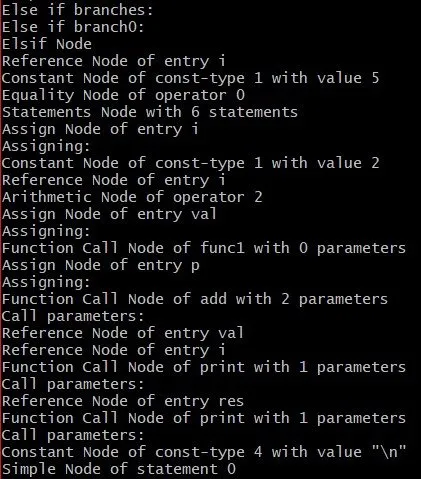You can see that the various function calls of the else if branch have been recognized, together with their calling parameters!

### Else part

Code:
``````...
else{
val = res[i];
print(res[i]);
print("\n");
p = p + 1;
}
...``````

Console: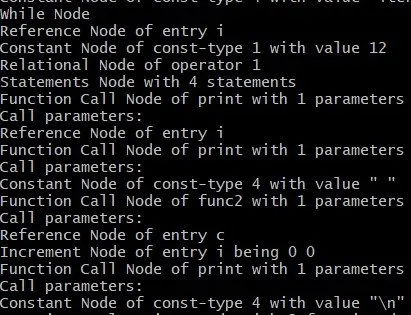You can see that the various function calls of the else branch have been recognized!

### While and Print

Code:
``````...
while(i < 12){
print(i);
print(" ");
func2(c);
i++;
}
print("\n");
...``````

Console:You can see that the various function calls of the while branch and the print statement, have all been recognized perfectly!

### Function func2

Code:
``````...
void func2(char c){
char *s;
*s = c;
print(*s);
}
...``````

Console: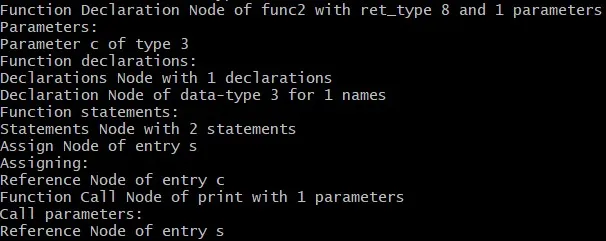You can see that the function call of "print" inside of the function declaration of "func2", has been recognized as it should!

## RESOURCES

### References:

No references, just using code that I implemented in my previous articles.

### Images:

All of the images are custom-made!

## Final words | Next up on the project

And this is actually it for today's post! I hope that I explained everything as much as I needed to, meaning that you learned something out of it.
Next up on this series are:
• Semantic analysis (using even more action rules in Bison)
• Machine Code generation (MIPS Assembly)
Which are all topics that will need more than one article to complete. Also, note that we might also get into Optimizations later on, or could even extend the Language by adding complex datatypes (structs and unions), more rules etc.
So, see ya next time!

## GitHub Account:

https://github.com/drifter1Keep on drifting! ;)

H2
H3
H4
3 columns
2 columns
1 column